• WGS84坐标间距离计算，WGS84转火星坐标gcj02，火星坐标转百度坐标BD09. function gcj02ToBd09(gg_lat, gg_lon: double): TLaLoDegPoint; //火星坐标系 (GCJ-02) 与百度坐标系 (BD-09) 的转换算法 将 GCJ-02 坐标转换...
• 项目中使用Echarts 地图，该地图百度已经停止维护了。由于项目需求，新增了许多行政区划区， 比如：高新区、经济开发区等 都...而我们只有wgs84的投影坐标文件。如果不加以转换则会造成严重的失准，故需要进行转化。
• WGS84转google坐标WGS84/GCJ02/BD09与墨卡托转换；地球坐标系 (WGS-84) 到火星坐标系 (GCJ-02) 的转换算法；WGS-84 到 GCJ-02 的转换（即 GPS 加偏）算法 火星坐标与地图坐标转换 C#版
• 可以支持大批量的文件进行WGS84坐标转北京54坐标坐标转换。内附范例数据格式，按照格式中的要求编辑即可批转换。
• 摄影测量 坐标转换 用MATLAB编写的坐标转换程序
• 坐标转换的小工具，经纬度转换成西安80坐标系，中央经线118.5
• WGS84转UTM坐标系，GPS坐标转大地坐标，Delphi实例源码。
• 将带有经度、纬度(WGS84坐标系)坐标的文本(*.txt)转换成指定投影坐标系的shp文件并计算x,y坐标。 环境和工具: WIN10、QGIS2.16、带有经纬度坐标的文本。格式如下图： 1、创建矢量图层 打开QGIS,选择Layer--&...
需求:
将带有经度、纬度(WGS84坐标系)坐标的文本(*.txt)转换成指定投影坐标系的shp文件并计算x,y坐标。
环境和工具:
WIN10、QGIS2.16、带有经纬度坐标的文本。格式如下图：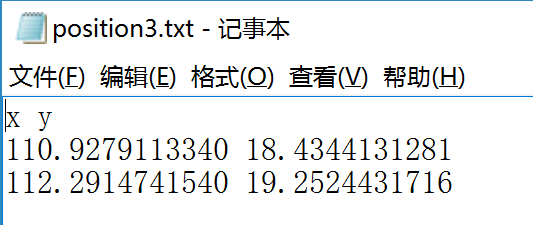1、创建矢量图层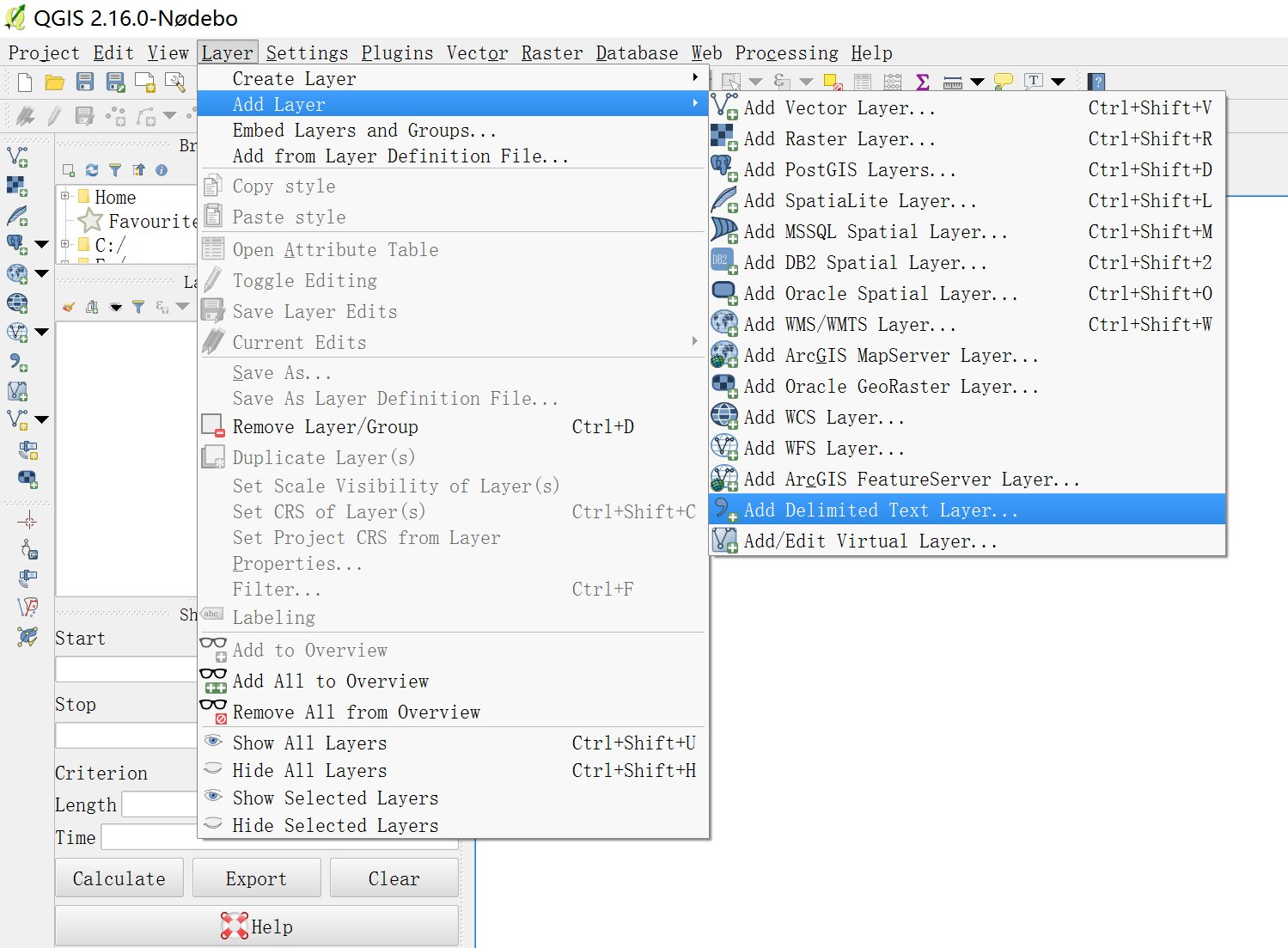2、导入文本坐标
弹出创建图层对话框，选择带有坐标的文本文件，选择自定自定义分割符(Custom delimiters),选择空格(Space),在X field和 Yfield下拉框中选择对应的x、y坐标，然后点击确定，如下图所示：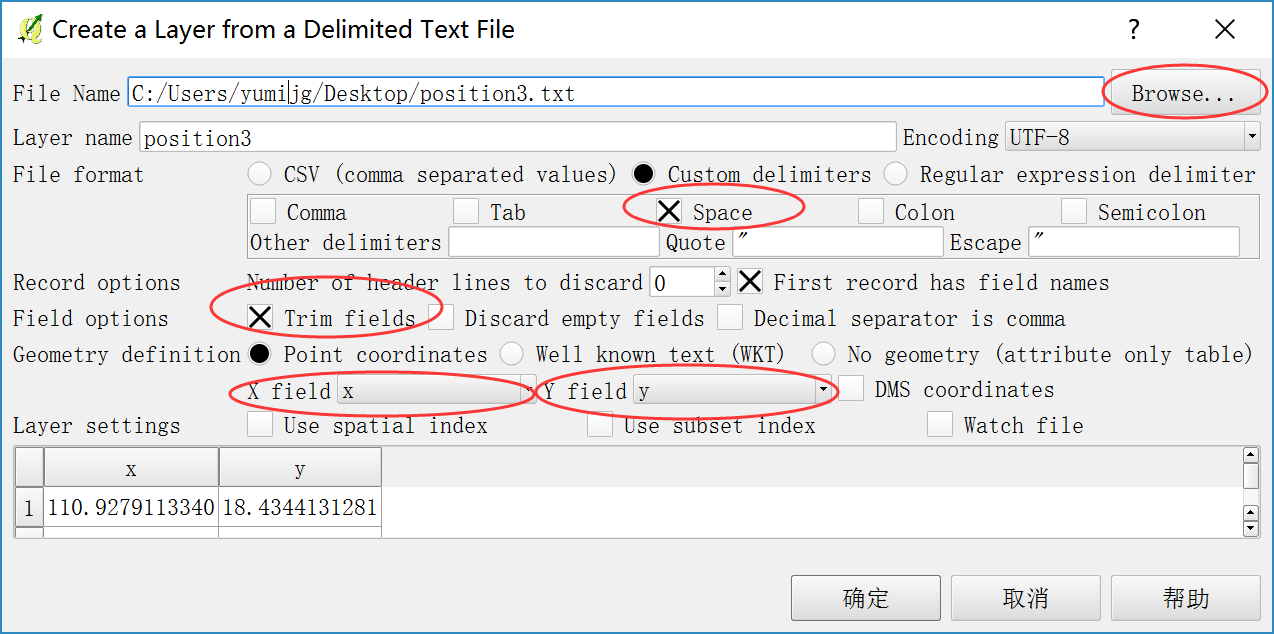3、选择坐标系
点击确定之后需要选择坐标系，这里选择WGS 84地理坐标系 在Filter搜索框中输入：WGS 84 选择EPSG:4326的坐标系，如下图所示：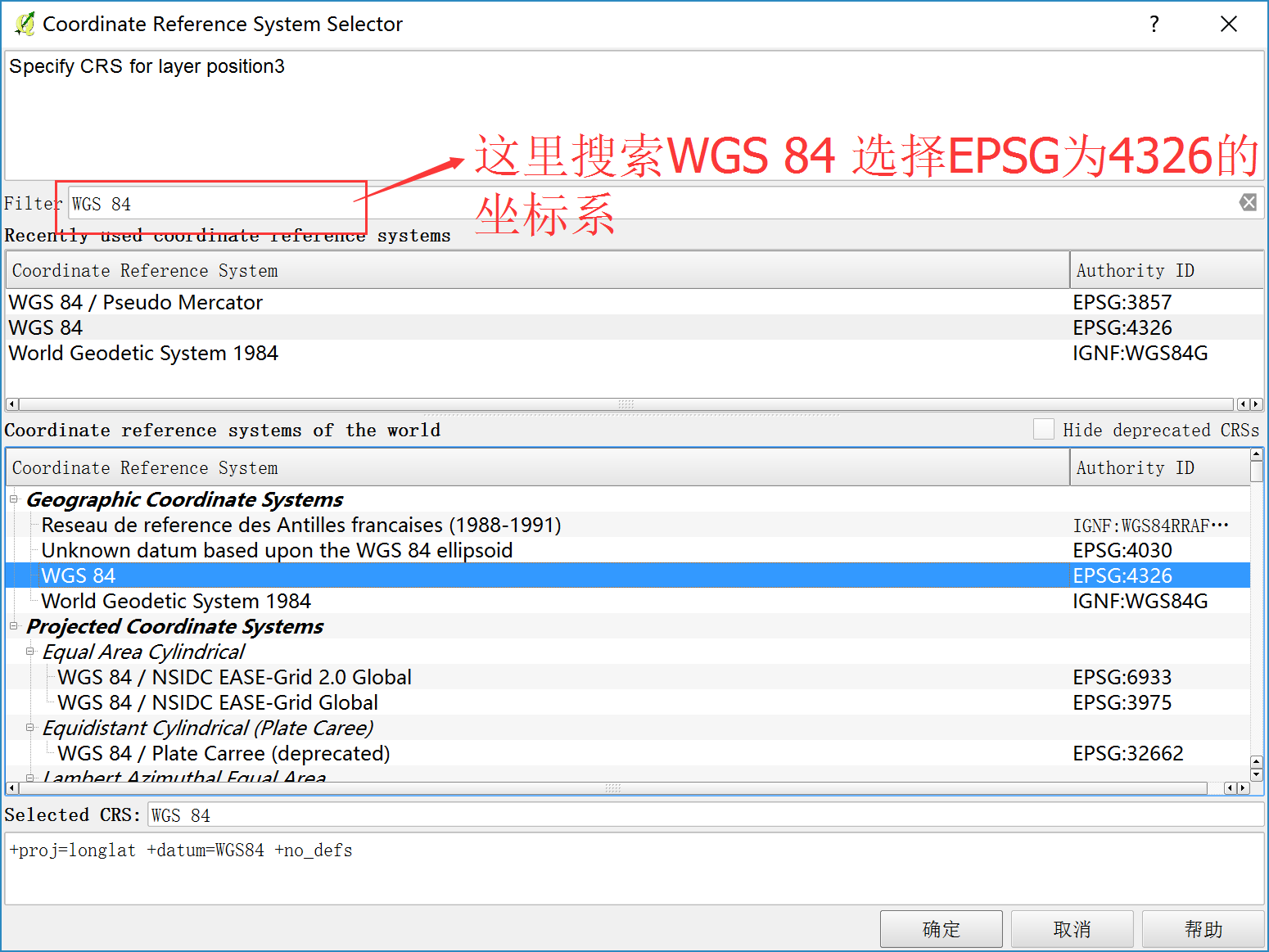4、另存矢量图层到本地计算机
这样数据就被导入进来了，然后另存数据，选中导入的图层，右键，选择Save　As，如下图所示：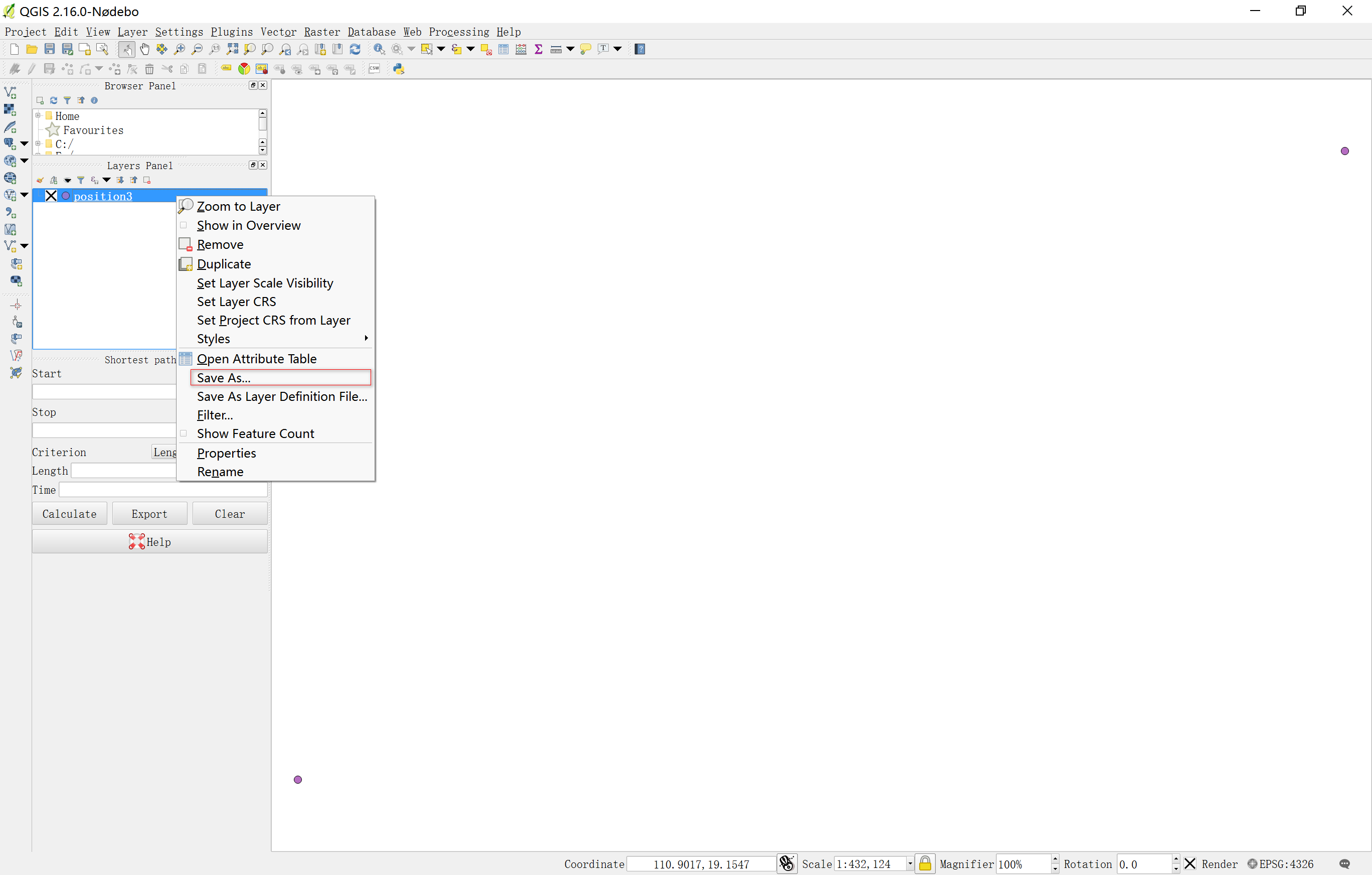5、设置导出图层参数
打开另存为对话框，这里导出的格式为ShapeFile。填写好文件路径和坐标系之后，点击确定就可以导出了，导出后文件会自动加载到QGIS中，如下图所示：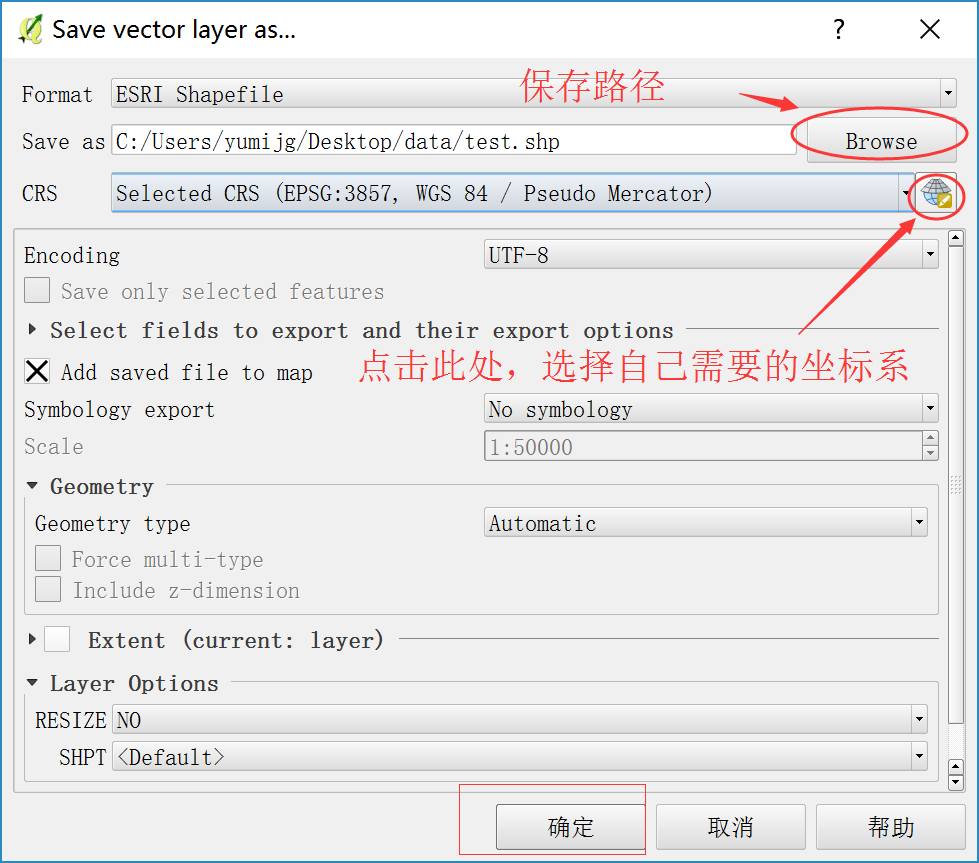6、打开属性表
右键打开shp图层的属性表，如下图所示：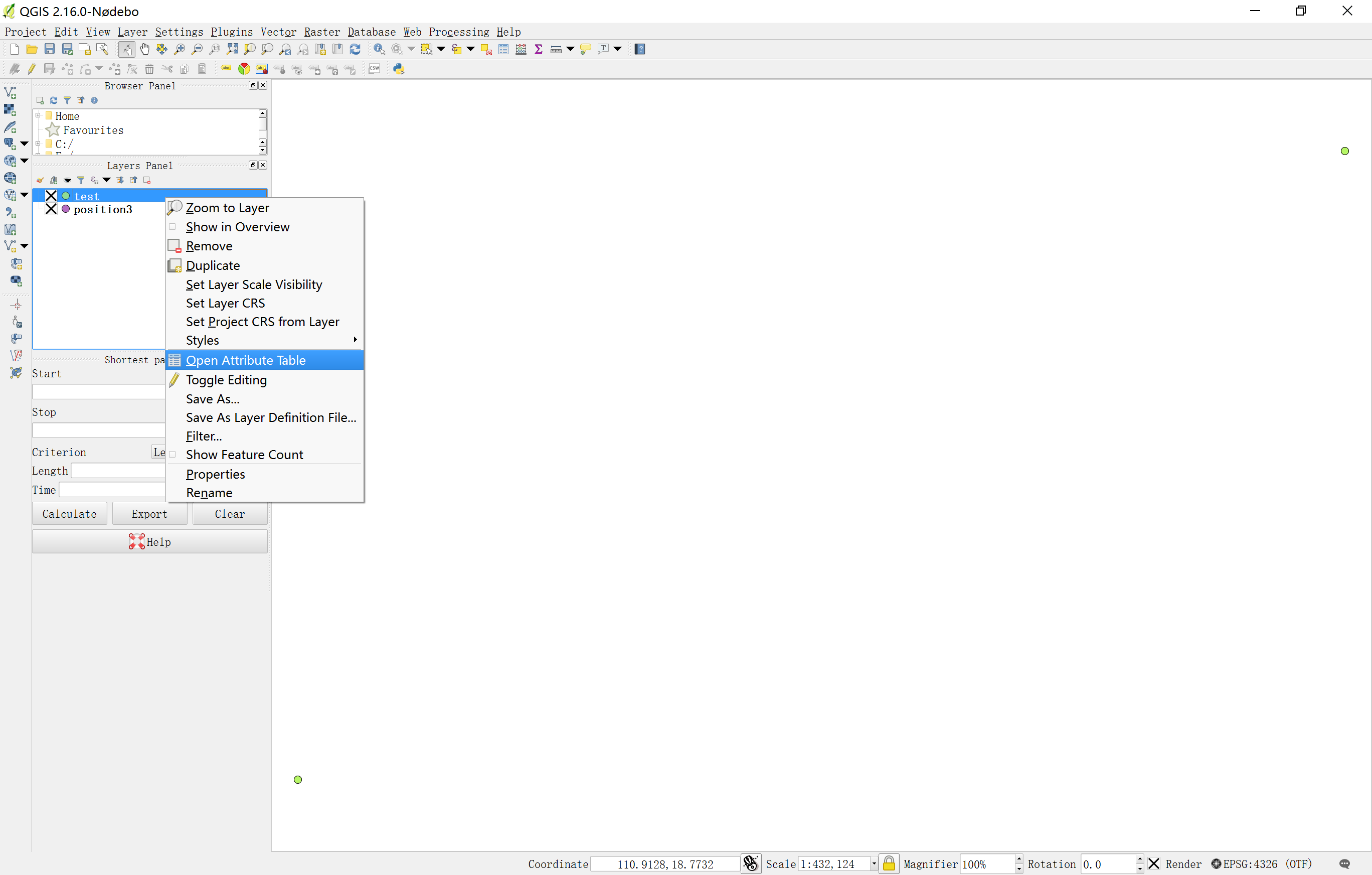7、打开字段计算器
打开属性表后开始计算X、Y坐标，点击属性表上面的:字段计算器(Calculator),如下图所示: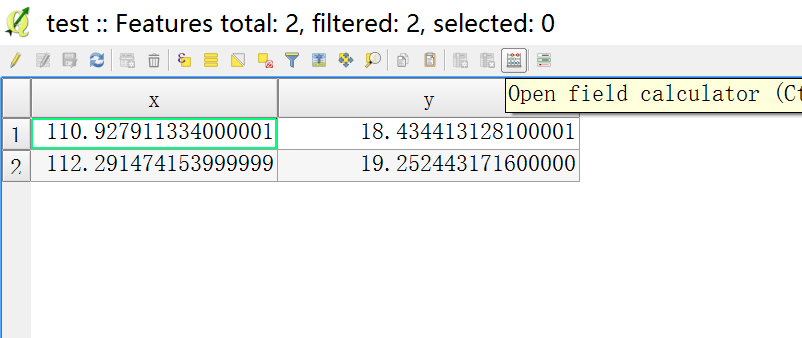8、计算X投影坐标
打开字段计算器后开始计算要素的投影坐标，选择Create a new field,输入字段名称，字段类型和精度之后、在row_number目录树中选择Geometry节点下面的\$x用来计算要素的x坐标。双击，将计算公式添加到左侧的计算框中，计算框下方会出现输出预览(Output preview),来查看输出结果是否正确，如果出现红色字体提示则出现了计算错误。然后点击确定即可得到计算结果。如下图所示：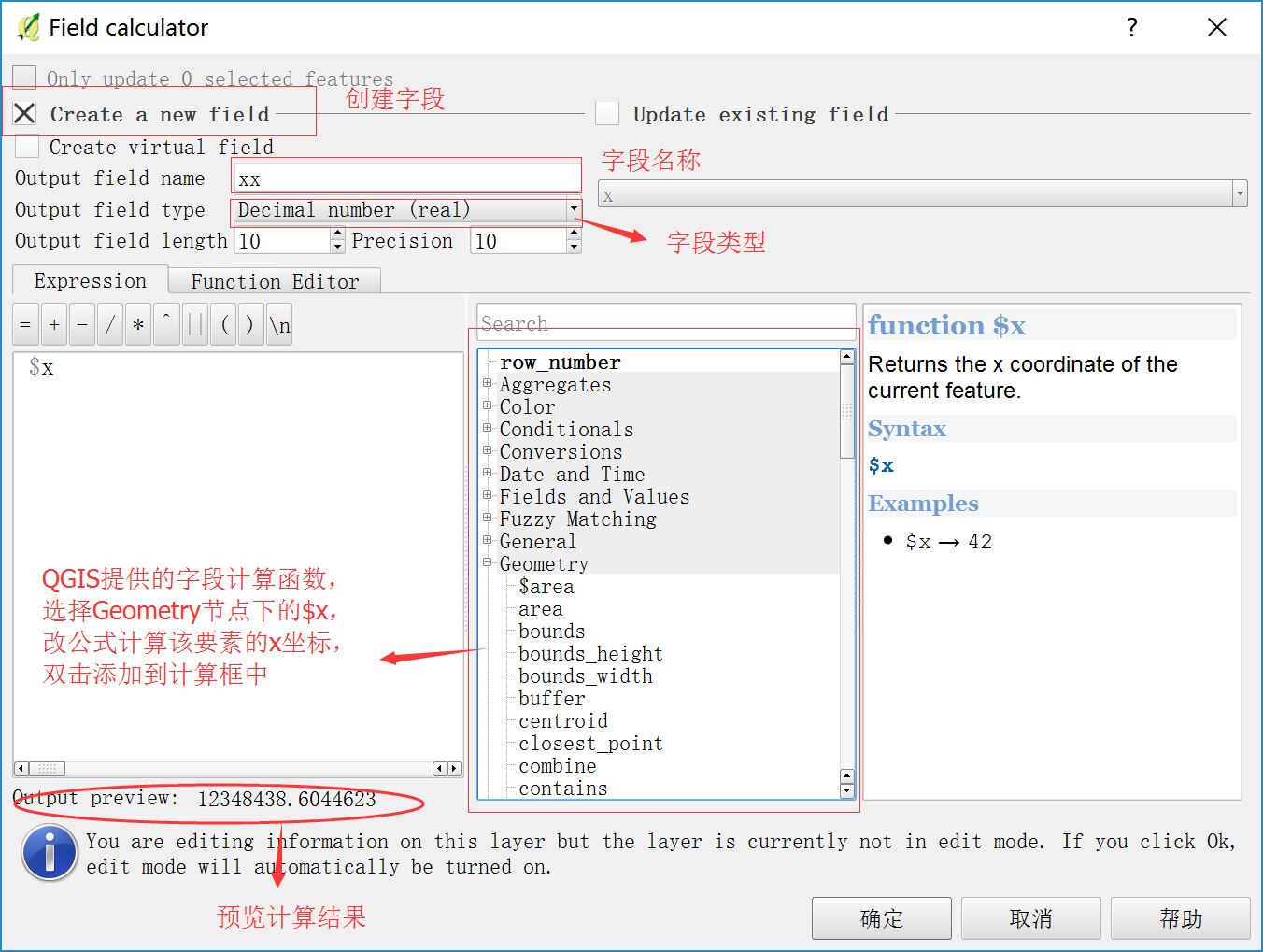计算结果如下图所示：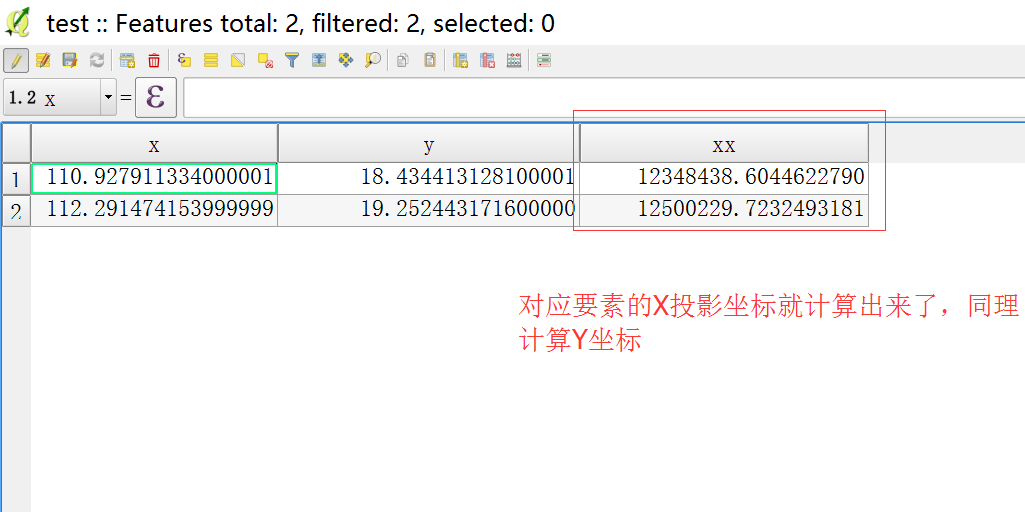9、计算Y投影坐标
计算Y坐标同理，如下图所示: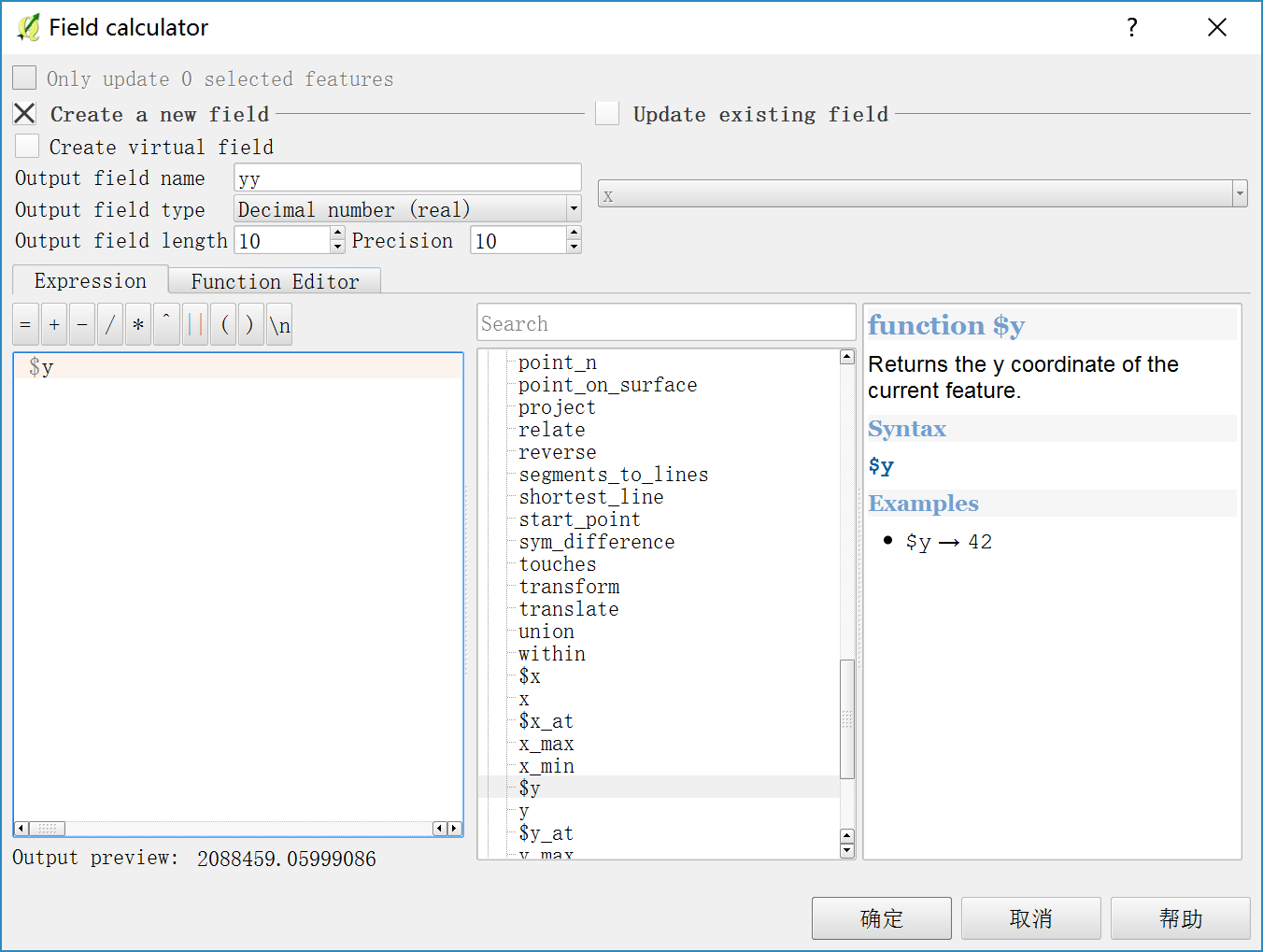PS:
这里说一下同样是x,y,为什么坐标不一样。
因为他们使用的坐标系不一样，文本里面的经纬度使用的是WGS 1984 地理坐标系，也是球面坐标系。而转换后的坐标系是墨卡托(Web Mercator)投影坐标系，是平面坐标系。他是将球面坐标经过一定的投影规则转化成平面坐标的。
想更加深入的了解坐标系可以自行百度,或者浏览以下博客：
Web Mercator 公开的小秘密
投影坐标系与地理坐标系的区别
地理坐标系、大地坐标系与地图投影与重投影详解
来源:https://www.cnblogs.com/GIScore/p/6525024.html
展开全文• WGS84-地方坐标转换 文档 关于GPS控制网WGS84平差坐标向地方独立坐标系的
• 经纬度坐标系之间相互转换，主要有wgs84坐标系、火星坐标系(国测局)、百度坐标系。用户只需通过一个html网页即可做到经纬度互，方便快捷高效，强力推荐呐~。
• wx.getLocation({ type: 'wgs84', //wgs84 success: (res) => { var lat = res.latitude var lng = res.longitude console.log(l...
wx.getLocation({
type: 'wgs84', //wgs84
success: (res) => {
var lat = res.latitude
var lng = res.longitude
console.log(lat + "||latitude" );
console.log(lng + "||longitude");
// wgs84转百度坐标系
var ssws=that.wgs84togcj02(lng, lat)
ssws = that.gcj02tobd09(ssws  , ssws )
//解决定位偏移
var ssssss1 = ssws - 0.000160
var ssssss2 = ssws - 0.000160

that.setData({ latitude: ssssss1.toFixed(6), longitude: ssssss2.toFixed(6) })
that.setData({
jd: ssssss2.toFixed(6),
wd: ssssss1.toFixed(6)
})
}
})
  //地图定位精确方法

/**
* WGS84转GCj02
* @param lng
* @param lat
* @returns {*[]}
*/

wgs84togcj02:function (lng, lat) {
var that=this

var x_PI = 3.14159265358979324 * 3000.0 / 180.0;
var PI = 3.1415926535897932384626;
var a = 6378245.0;
var ee = 0.00669342162296594323;
if (that.out_of_china(lng, lat)) {
return [lng, lat]
}
else {
var dlat = that.transformlat(lng - 105.0, lat - 35.0);
var dlng = that.transformlng(lng - 105.0, lat - 35.0);
var radlat = lat / 180.0 * PI;
magic = 1 - ee * magic * magic;
var sqrtmagic = Math.sqrt(magic);
dlat = (dlat * 180.0) / ((a * (1 - ee)) / (magic * sqrtmagic) * PI);
dlng = (dlng * 180.0) / (a / sqrtmagic * Math.cos(radlat) * PI);
var mglat = lat + dlat;
var mglng = lng + dlng;
return [mglng, mglat]
}
},
/**
* 火星坐标系 (GCJ-02) 与百度坐标系 (BD-09) 的转换
* 即谷歌、高德 转 百度
* @param lng
* @param lat
* @returns {*[]}
*/
gcj02tobd09:function(lng, lat) {
var that = this
var x_PI = 3.14159265358979324 * 3000.0 / 180.0;
var PI = 3.1415926535897932384626;
var a = 6378245.0;
var ee = 0.00669342162296594323;
var z = Math.sqrt(lng * lng + lat * lat) + 0.00002 * Math.sin(lat * x_PI);
var theta = Math.atan2(lat, lng) + 0.000003 * Math.cos(lng * x_PI);
var bd_lng = z * Math.cos(theta) + 0.0065;
var bd_lat = z * Math.sin(theta) + 0.006;
return [bd_lng, bd_lat]
},
transformlat:function (lng, lat) {
var x_PI = 3.14159265358979324 * 3000.0 / 180.0;
var PI = 3.1415926535897932384626;
var a = 6378245.0;
var ee = 0.00669342162296594323;
var ret = -100.0 + 2.0 * lng + 3.0 * lat + 0.2 * lat * lat + 0.1 * lng * lat + 0.2 * Math.sqrt(Math.abs(lng));
ret += (20.0 * Math.sin(6.0 * lng * PI) + 20.0 * Math.sin(2.0 * lng * PI)) * 2.0 / 3.0;
ret += (20.0 * Math.sin(lat * PI) + 40.0 * Math.sin(lat / 3.0 * PI)) * 2.0 / 3.0;
ret += (160.0 * Math.sin(lat / 12.0 * PI) + 320 * Math.sin(lat * PI / 30.0)) * 2.0 / 3.0;
return ret
},

transformlng:function (lng, lat) {
var x_PI = 3.14159265358979324 * 3000.0 / 180.0;
var PI = 3.1415926535897932384626;
var a = 6378245.0;
var ee = 0.00669342162296594323;
var ret = 300.0 + lng + 2.0 * lat + 0.1 * lng * lng + 0.1 * lng * lat + 0.1 * Math.sqrt(Math.abs(lng));
ret += (20.0 * Math.sin(6.0 * lng * PI) + 20.0 * Math.sin(2.0 * lng * PI)) * 2.0 / 3.0;
ret += (20.0 * Math.sin(lng * PI) + 40.0 * Math.sin(lng / 3.0 * PI)) * 2.0 / 3.0;
ret += (150.0 * Math.sin(lng / 12.0 * PI) + 300.0 * Math.sin(lng / 30.0 * PI)) * 2.0 / 3.0;
return ret
},

/**
* 判断是否在国内，不在国内则不做偏移
* @param lng
* @param lat
* @returns {boolean}
*/
out_of_china:function (lng, lat) {
return (lng < 72.004 || lng > 137.8347) || ((lat < 0.8293 || lat > 55.8271) || false);
}

部分参考https://blog.csdn.net/yzyssg1/article/details/76120617

开发工具定位和手机的定位相差很大 手机调试准确一些
如果你觉得这篇内容对你挺有启发请点赞+关注
展开全文• WGS84与北京54坐标转换研究，国际与国内主要应用坐标系统的转换研究
• GIS中关于WGS84与北京54坐标系之间的转换，
• 一、UTM坐标简介 二、WGS84简介 三、WGS84 UTM 四、UTM如何转换为WGS84坐标

目录
一、UTM坐标简介
二、WGS84简介
三、WGS84 转 UTM
四、UTM如何转换为WGS84坐标
补充 UTM

一、UTM坐标简介
像橘子一样均匀地切成60个称为“区域”的部分，展平这些区域中的每个区域，通用横轴Mercator（UTM）投影；
可以这样看：UTM区域是地球的6°区域，因为圆具有360°，这意味着地球上有60个UTM区域。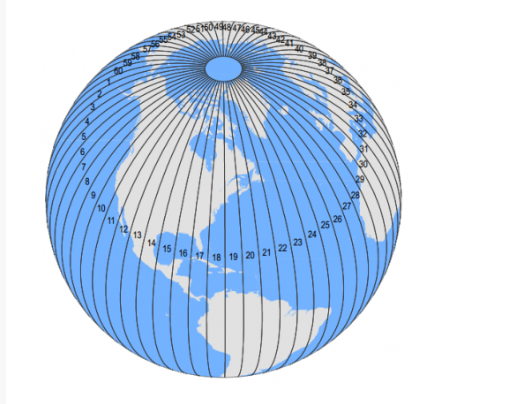比如：中国东部地区属于UTM Zone 50N，可以到官网找到目标地区的编号
官网：http://www.dmap.co.uk/utmworld.htm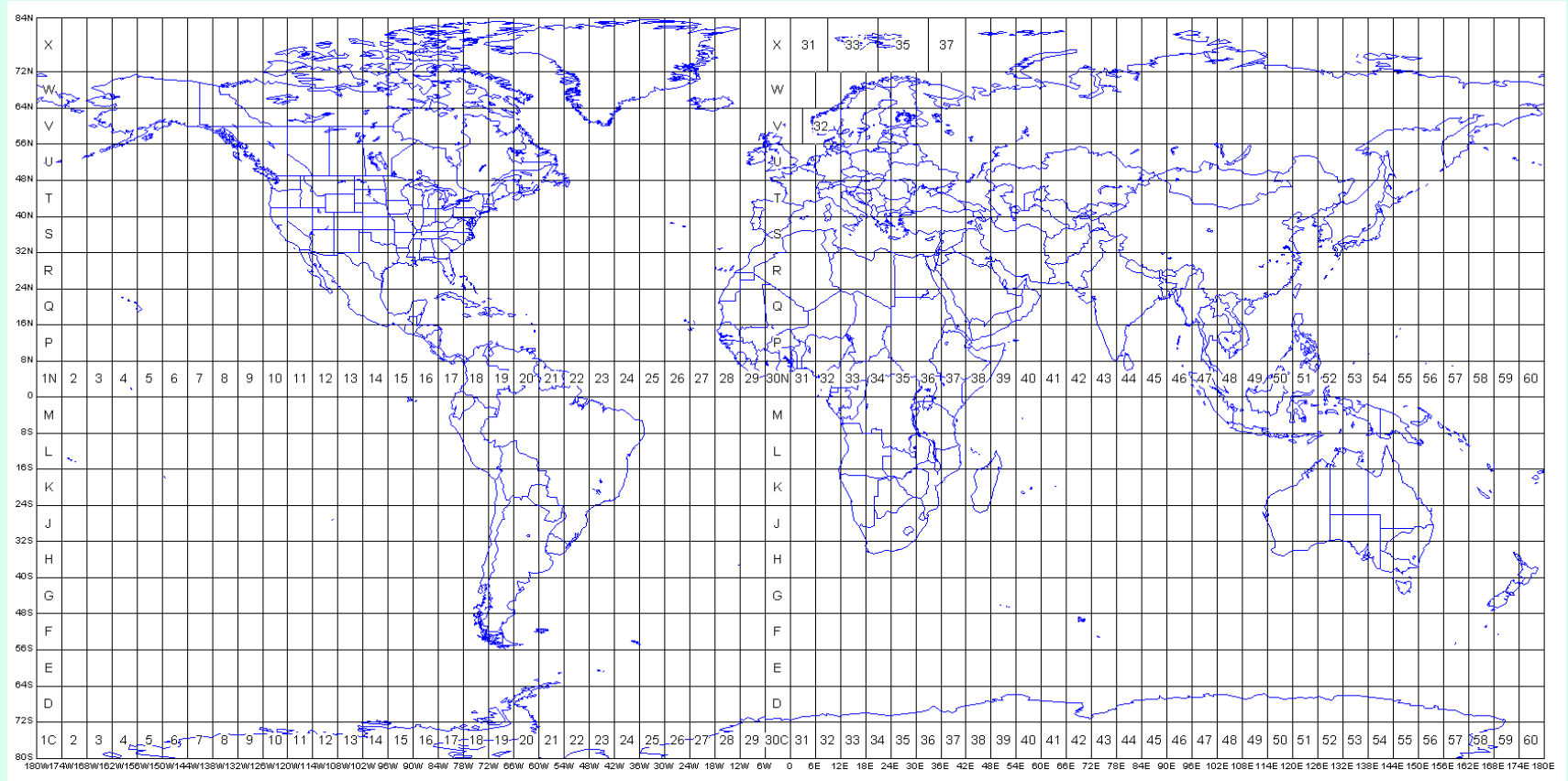原理图：
X轴：指向东边、Y轴：指向北边、Z轴：指向天顶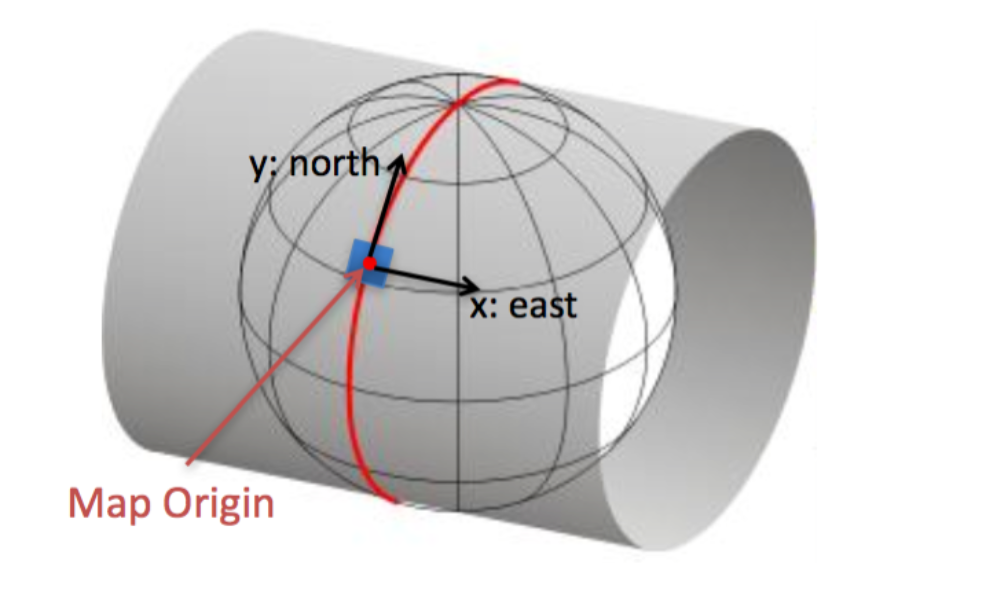本坐标系采用三维直角坐标系来描述地球表面，实际应用较为困难，因此一般使用简化后的二维投影坐标系来描述。
想了解细节的的朋友可以去看看：
如何读取UTM坐标
http://geokov.com/Education/utm.aspx
如果想进一步搞清楚原理，文档后面有补充。

二、WGS84简介
WGS84：世界大地测量系统  其英文是：World Geodetic System；该误差被认为小于2厘米。
WGS84是GPS的标准配置，全球定位系统使用WGS84作为参考坐标系。
它使用地球的中心质量作为坐标原点，参考椭球，标准坐标系，高度数据和大地水准面组成。（包括：经度，纬度，海拔高度）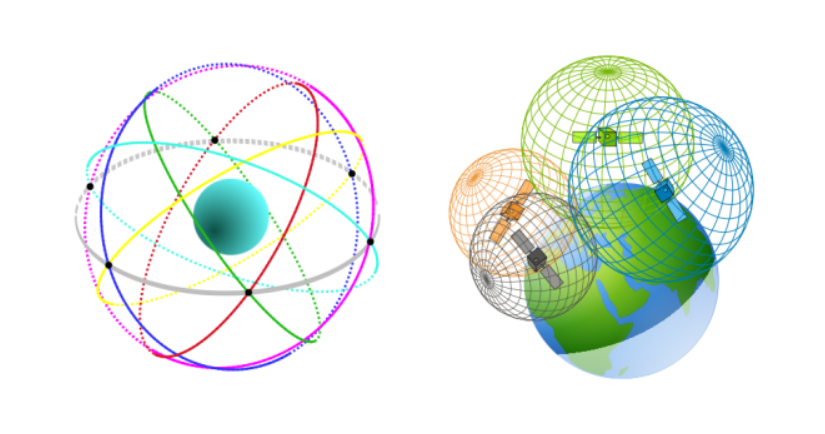定义的原理可以看看下图：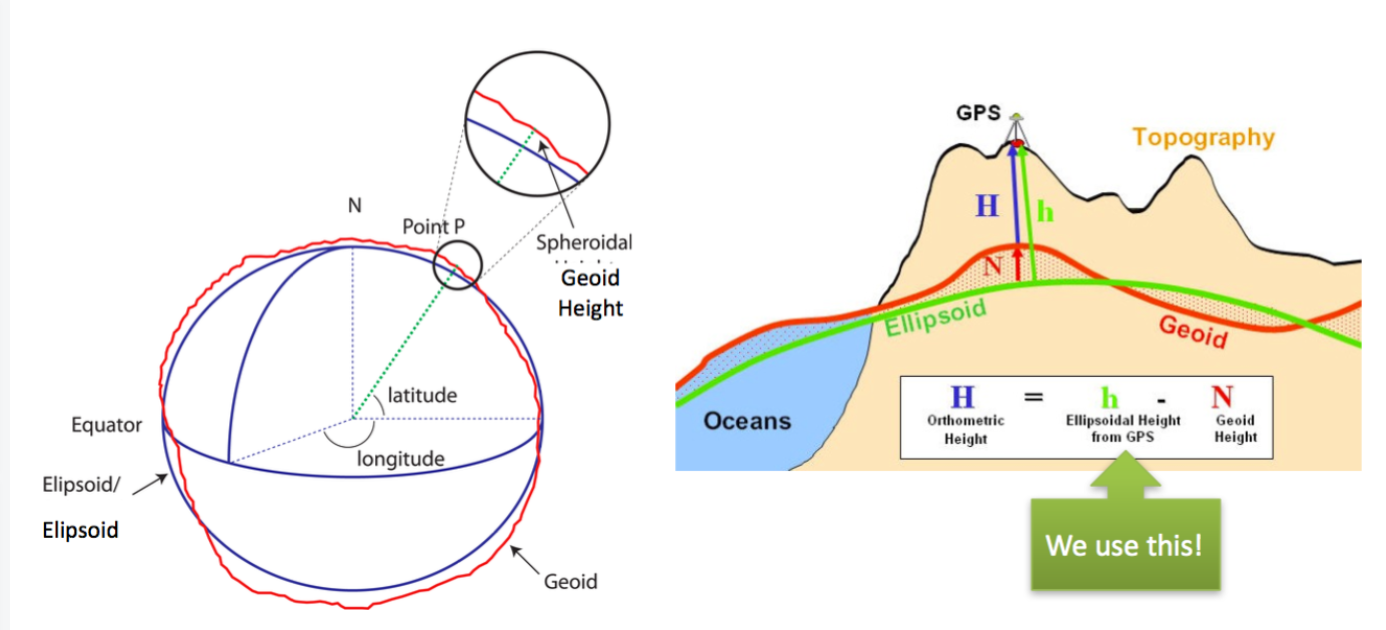核心留意几个点：纬度（latitude）、经度（longitude）和海拔（elevation）
想了解细节的的朋友可以去看看：https://www.linz.govt.nz/data/geodetic-system/datums-projections-and-heights/geodetic-datums/world-geodetic-system-1984-wgs84
https://confluence.qps.nl/qinsy/latest/en/world-geodetic-system-1984-wgs84-182618391.html

三、WGS84 转 UTM
请直接看我这篇博客：WGS84 与 UTM 互转（Python代码版）​​​​​​​
本文不讲原理，只分享实践思路和代码。基于Python语言，使用pyproj库进行WGS 和UTM的转换。

四、UTM如何转换为WGS84坐标
方案一：
在线UTM到经度转换器（WGS84）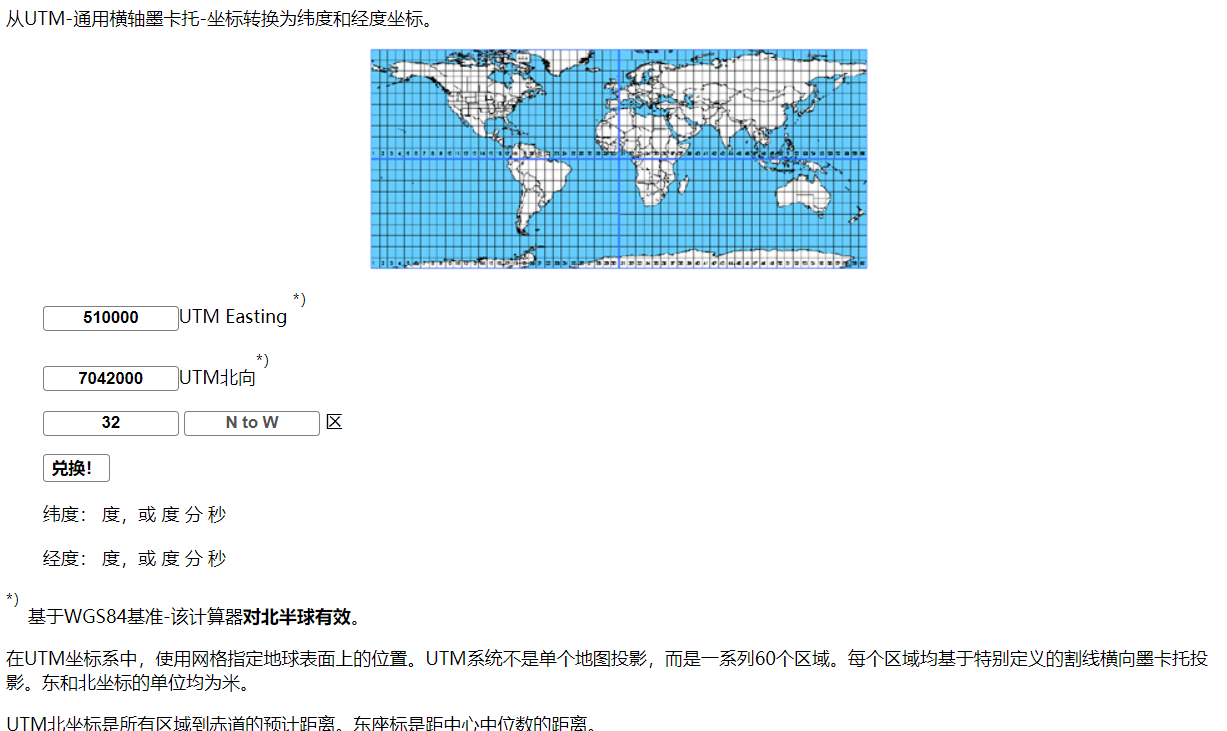https://www.engineeringtoolbox.com/utm-latitude-longitude-d_1370.html

方案二：
原地址：https://www.cnblogs.com/kennyhr/p/9353647.html
例如：中国东部地区属于UTM Zone 50N 查找UTM 50N 的 EPSG 执行标准 https://epsg.io/?q=UTM+zone+50N WGS 84 / UTM zone 50N EPSG:32650
>>> from pyproj import Proj,transform
>>> WGS84 = Proj(init='EPSG:4326')
>>> p = Proj(init="EPSG:32650")
>>> x,y = 526434.351935, 3522210.609046
>>> transform(p, WGS84, x, y)
(117.27936202563953, 31.835267862875163)

希望对你有帮助。

补充 UTM
UTM 使用直立圆柱体进行地图投影。横轴墨卡托将圆柱体放在侧面（旋转90°），如下图所示–这就是“横轴”一词的推导方式。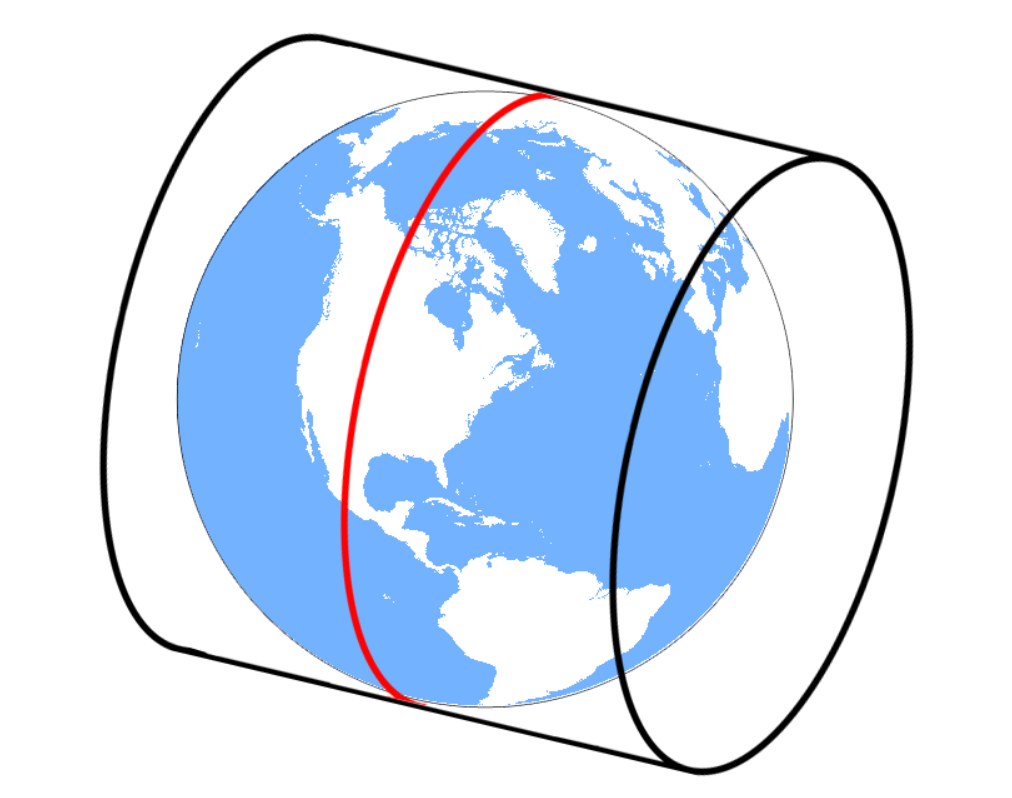但是，通用横轴墨卡托将每个UTM区域将此圆柱放置60次。
这意味着所有60个楔形块都用横向圆柱体弄平了。每次使用不同的子午线作为中心线稍微旋转时。
UTM使用正割圆柱体，该圆柱体沿着平行于中央子午线的两个小圆圈与椭圆体相交。这意味着子午线的尺度在南北恒定。但是规模沿东西方向平行变化。这两个小圆圈位于赤道中央子午线以西180公里。小圆圈的比例因子为1，这意味着在椭球体上100米的距离在地图投影上将是相同的。
UTM网格区域的中心线的比例因子为0.9996。这意味着在椭球上100米的距离在地图上将为99.96米。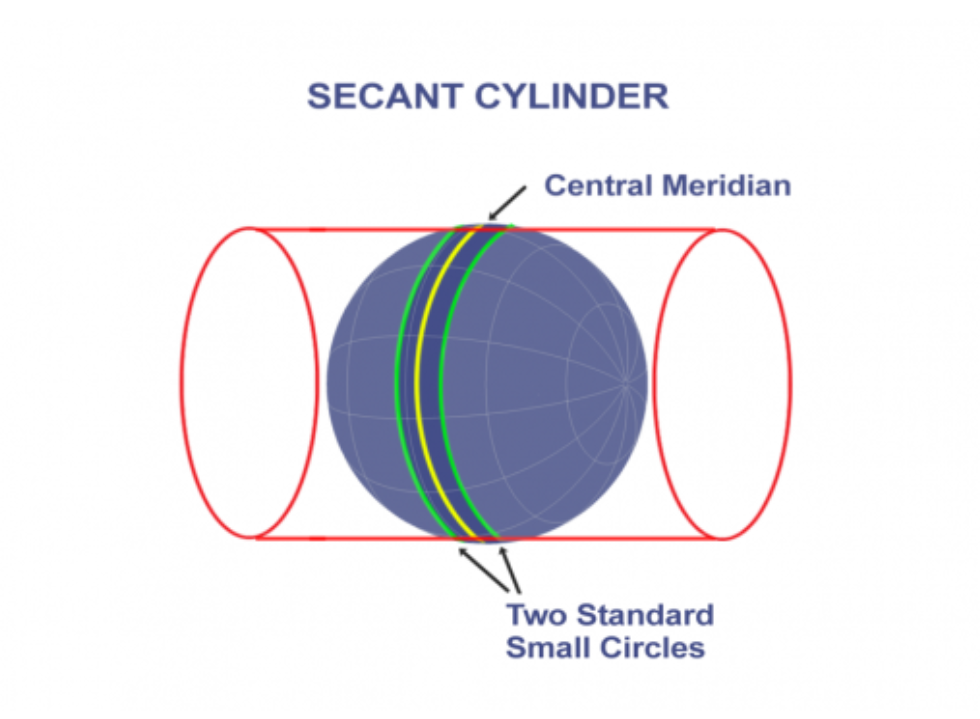本文只提供参考学习，谢谢。
展开全文转换
•C语言
• WGS84经纬度坐标到北京54高斯投影坐标的转换
• WGS84经纬度坐标与北京54坐标或者西安80坐标的关系
• 实现大地坐标与空间直角坐标和平面坐标的相互转换以及不同参考椭球的坐标系转换，内含8454坐标转换示例！
• 本工具为excel进行开发，针对北京54wgs84坐标系的相互转换功能。精准小工具
• 基于MATLAB的WGS84坐标向北京54坐标转换.pdf
• #include "stdafx.h" #include <math.h> #define PI 3.141592653 ...该程序根据GPS.G1-X-00006.pdf文档，实现了WGS84和ECEF坐标的转换 */ void LLAtoECEF(double latitude, double longitude, double...

#include "stdafx.h"
#include <math.h>

#define  PI  3.141592653
/*
该程序根据GPS.G1-X-00006.pdf文档，实现了WGS84和ECEF坐标的转换
*/

void LLAtoECEF(double latitude, double longitude, double height, double &X, double &Y, double &Z)
{
double a = 6378137;
double b = 6356752.314245;// b=(1-f)
double E = (a*a - b*b)/(a*a);

double COSLAT = cos(latitude*PI/180);
double SINLAT = sin(latitude*PI/180);
double COSLONG = cos(longitude*PI/180);
double SINLONG = sin(longitude*PI/180);

double N = a /(sqrt(1 - E*SINLAT*SINLAT));
double NH = N + height;

X = NH * COSLAT * COSLONG;
Y = NH * COSLAT * SINLONG;
Z = (b*b*N/(a*a) + height) * SINLAT;
}

void ECEFtoLLA(int lx, int ly, int lz)
{
double      x, y, z;
double      a, b, f, e, e1;
double      Longitude;
double      Latitude;
double      Altitude;
double      p, q;
double      N;
x = (double)lx ;
y = (double)ly ;
z = (double)lz ;
a = (double)6378137.0;
b = (double)6356752.31424518;
f = 1.0 / (double)298.257223563;
e = sqrtl(((a * a) - (b * b)) / (a * a));
e1 = sqrtl(((a * a) - (b * b)) / (b * b));
p = sqrtl((x * x) + (y * y));
q = atan2l((z * a), (p * b));
Longitude = atan2l(y, x);
Latitude = atan2l((z + (e1 * e1) * b * powl(sinl(q), 3)), (p - (e * e) * a * powl(cosl(q), 3)));
N = a / sqrtl(1 - ((e * e) * powl(sinl(Latitude), 2)));
Altitude = (p / cosl(Latitude)) - N;
Longitude = Longitude * 180.0 / PI;
Latitude = Latitude  * 180.0 / PI;
Latitude = (double)Latitude;
Longitude = (double)Longitude;
Altitude = (double)Altitude;
}

int _tmain(int argc, _TCHAR* argv[])
{
double latitude = 22.21555549969;
double longitude = 113.367229848;
double h = 0.5;
double XX = 0.0;
double YY = 0.0;
double ZZ = 0.0;
LLAtoECEF(latitude, longitude, h, XX, YY, ZZ);
ECEFtoLLA(XX, YY, ZZ);
return 0;
}

注意
当前程序对于海拔高度的转换，出现问题，需要重新调整

本文转自fengyuzaitu 51CTO博客，原文链接：http://blog.51cto.com/fengyuzaitu/1892212，如需转载请自行联系原作者


展开全文• WGS-84 到BJ-54 内含高斯正反算 大地坐标系与空间直角坐标转换 弧度度 七参数
• 提供百度坐标WGS84，高德、谷歌坐标WGS84坐标的转换，提供百度、高德、谷歌与WGS84、火星之间的转换...